## 2.2.2 Drift-Diffusion Transport Model

MAXWELL's equations can be used to derive POISSON's equation (2.14) and the current continuity equation (2.18), whereas the current relations cannot be derived from them.

The causes of current flow are manifold. One contribution is determined by OHM's law which connects the current density to the electric field. Other causes might be gradients of the carrier concentration or the temperatures of the lattice or the carriers, or gradients of material properties.

In semiconductors two separate particle systems are responsible for charge transport. The carriers of charge are electrons (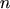) and holes (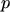) and their movement is responsible for the conduction2.2current density which can be written as the sum of two components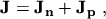(2.19)

where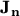and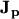are the electron and hole current density, respectively.

Subsections

M. Gritsch: Numerical Modeling of Silicon-on-Insulator MOSFETs PDF